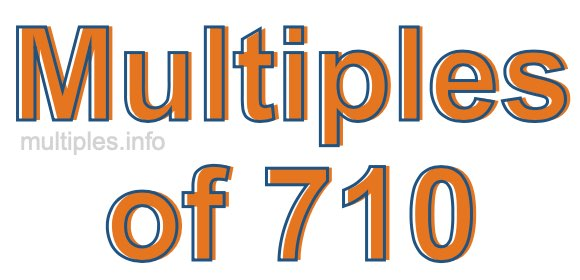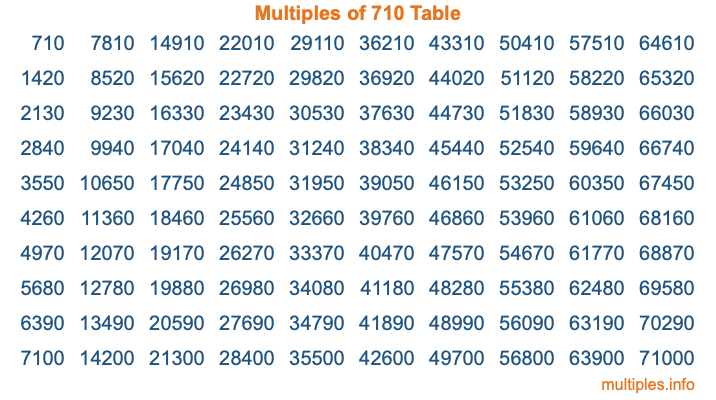Multiples of 710Welcome to the Multiples of 710 page. Here we will first teach you everything you will ever need to know about the multiples of 710, and then give you a study guide summary of everything we taught you to make sure you remember it all. Use this page to look up facts and learn information about the multiples of 710. This page will make you a multiples of seven hundred ten expert!

Definition of Multiples of 710
Multiples of 710 are all the numbers that when divided by 710 equal an integer. Each of the multiples of 710 are called a multiple. A multiple of 710 is created by multiplying 710 by an integer.

Therefore, to create a list of multiples of 710, you start with 1 multiplied by 710, then 2 multiplied by 710, then 3 multiplied by 710, and so on for as long as you want. Thus, the list of the first five multiples of 710 is 710, 1420, 2130, 2840, and 3550. To see a larger list of multiples of 710, see the printable image of Multiples of 710 further down on this page. We also have a category where you can choose any nth multiple of 710.

Multiples of 710 Checker
The Multiples of 710 Checker below checks to see if any number of your choice is a multiple of 710. In other words, it checks to see if there is any number (integer) that when multiplied by 710 will equal your number. To do that, we divide your number by 710. If the the quotient is an integer, then your number is a multiple of 710.

Is  a multiple of 710?

Least Common Multiple of 710 and ...
A Least Common Multiple (LCM) is the lowest multiple that two or more numbers have in common. This is also called the smallest common multiple or lowest common multiple and is useful to know when you are adding our subtracting fractions. Enter one or more numbers below (710 is already entered) to find the LCM.

Check out our LCM Calculator if you need more details about the Least Common Multiple or if you need the LCM for different numbers for adding and subtraction fractions.

nth Multiple of 710
As we stated above, 710 is the first multiple of 710, 1420 is the second multiple of 710, 2130 is the third multiple of 710, and so on. Enter a number below to find the nth multiple of 710.

th multiple of 710

Multiples of 710 vs Factors of 710
710 is a multiple of 710 and a factor of 710, but that is where the similarities end. All postive multiples of 710 are 710 or greater than 710. All positive factors of 710 are 710 or less than 710.

Below is the beginning list of multiples of 710 and the factors of 710 so you can compare:

Multiples of 710: 710, 1420, 2130, 2840, 3550, etc.

Factors of 710: 1, 2, 5, 10, 71, 142, 355, 710

As you can see, the multiples of 710 are all the numbers that you can divide by 710 to get a whole number. The factors of 710, on the other hand, are all the whole numbers that you can multiply by another whole number to get 710.

It's also interesting to note that if a number (x) is a factor of 710, then 710 will also be a multiple of that number (x).

Multiples of 710 vs Divisors of 710
The divisors of 710 are all the integers that 710 can be divided by evenly. Below is a list of the divisors of 710.

Divisors of 710: 1, 2, 5, 10, 71, 142, 355, 710

The interesting thing to note here is that if you take any multiple of 710 and divide it by a divisor of 710, you will see that the quotient is an integer.

Multiples of 710 Table
Below is an image of the first 100 multiples of 710 in a table. The table is in chronological order, column by column. The first column has the first ten multiples of 710, the second column has the next ten multiples of 710, and so on.The Multiples of 710 Table is also referred to as the 710 Times Table or Times Table of 710. You are welcome to print out our table for your studies.

Negative Multiples of 710
Although not often discussed or needed in math, it is worth mentioning that you can make a list of negative multiples of 710 by multiplying 710 by -1, then by -2, then by -3, and so on, to get the following list of negative multiples of 710:

-710, -1420, -2130, -2840, -3550, etc.

Multiples of 710 Summary
Below is a summary of important Multiples of 710 facts that we have discussed on this page. To retain the knowledge on this page, we recommend that you read through the summary and explain to yourself or a study partner why they hold true.

There are an infinite number of multiples of 710.

A multiple of 710 divided by 710 will equal a whole number.

710 divided by a factor of 710 equals a divisor of 710.

The nth multiple of 710 is n times 710.

The largest factor of 710 is equal to the first positive multiple of 710.

710 is a multiple of every factor of 710.

710 is a multiple of 710.

A multiple of 710 divided by a divisor of 710 equals an integer.

710 divided by a divisor of 710 equals a factor of 710.

Any integer times 710 will equal a multiple of 710.

Multiples of a Number
Here you can get the multiples of another number, all with the same attention to detail as we did for multiples of 710 on this page.

Multiples of
Multiples of 711
Did you find our page about multiples of seven hundred ten educational? Do you want more knowledge? Check out the multiples of the next number on our list!